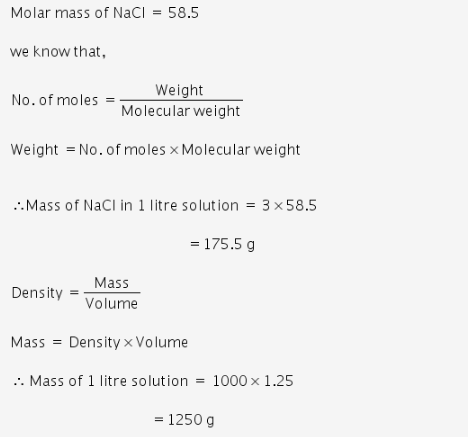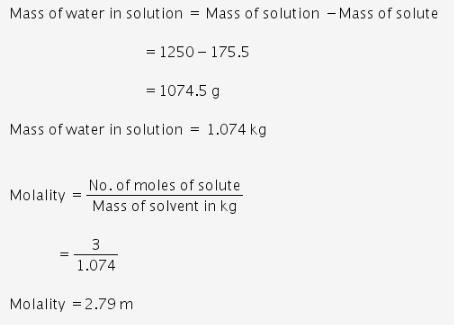# The density of 3M solution of NaCl is 1.25 g/mL. Calculate molality of the solution

The density of 3M solution of NaCl is 1.25 g/mL. Calculate molality of the solution

Given:
Density of solution = 1.25 g/mL
M =3 mol/litMolality of the solution is 2.79 m.